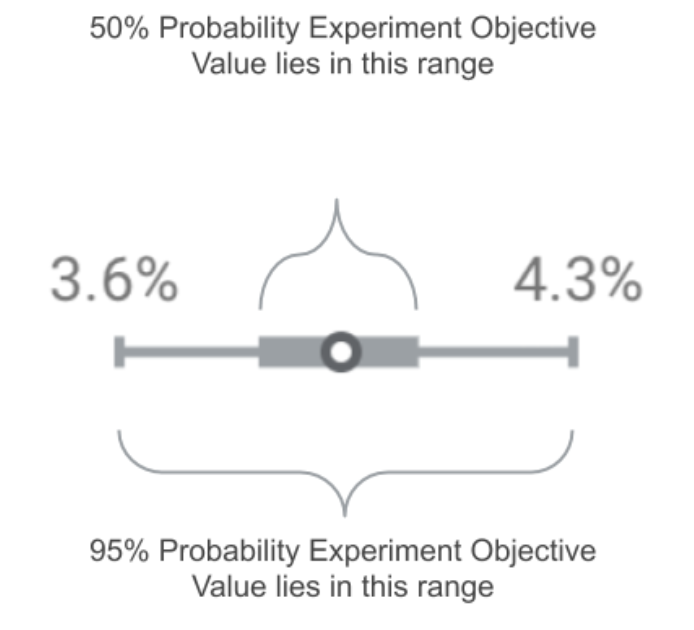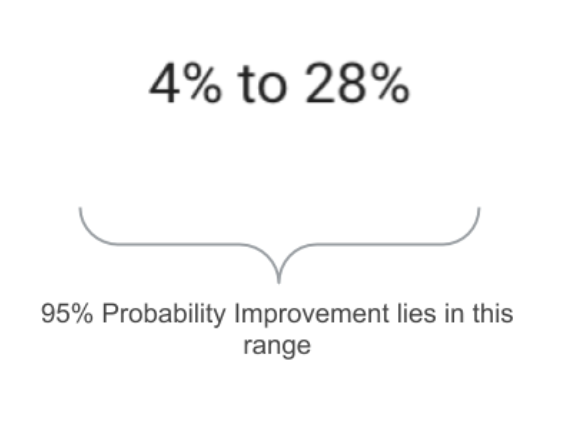# Optimize reports explained

## What is a credible interval?

Understanding the concept of a credible interval is critical in understanding what follows.

A credible interval is a range of possible values for the experiment objective you are trying to measure. An experiment doesn't know the true value of the objective because it sees only a sample of the site traffic. The range of possible values is based on this observed sample.

Credible interval: There is a X% chance that the true value is within the X% credible interval.

Examples

• 95% credible interval: There's a 95% chance that the true value of your objective is within the 95% credible interval.
• 50% credible interval: There's a 50% chance that the true value of your objective is within the 50% credible interval.

## How Optimize uses credible intervals

Optimize analysis shows credible intervals for two different things: modeled conversion rate (a.k.a. "modeled objective value") and modeled improvement. Although they're displayed differently, they're both credible intervals.

Optimize analysis shows both the 95% and 50% credible intervals. This means that with the outer range (the 95% credible interval) you can say that there's a 95% probability that the experiment objective is in the credible interval range.

The experiment Modeled Conversion Rate interval is displayed by Optimize as follows:Optimize Analysis makes the credible interval a symmetric range as shown in the diagram. This means that the remaining 5% probability is split evenly above and below the interval. So you can say that there is a 2.5% probability that the value of the experiment objective is less than the lower bound of the credible interval. And just as well, there is a 2.5% probability that the value of the experiment objective is more than the upper bound of the credible interval.

### For statistics fans

If you love doing math, you can take it even further. You can just as easily say there is a 22.5% probability that the true experiment objective value lies between the lower bound of the 95% credible interval and the lower bound of the 50% credible interval. But that may not be too interesting unless you love data and inferences like we do.

## Modeled improvement

Modeled improvement is the relative lift a variant may see over the baseline. This is also displayed as a credible interval, but in a slightly different format.The modeled improvement column in Optimize reports displays results at the 95% credible interval. The 50% credible interval is shown when you hover over the results. Optimize’s credible intervals provide a probability statement on the range of likely values in an experiment.

But wait this sounds like a confidence interval. What's the difference?

When used in frequentist methods, a confidence interval is a probability about whether the interval presented contains the value of the experiment objective (or improvement).

## Modeled objective rate

Modeled objective rate represents the long-run average of where Optimize expects the value of the specified objective to land. For example, if you have a revenue objective, Optimize is modeling the long-run average revenue per session. Or, for pageviews, the long-run average pageviews per session.

Because Optimize is modeling the long-run rate, there are two points that must be stressed:

First, if you deploy or update your site to reflect the winning variant, you may not immediately achieve the modeled conversion rate. It can take up to as long as you had the experiment running.

Second, Optimize assumes that the site traffic during the experiment is similar to what will happen after a variant is deployed. As you deploy or update the site based on test results, ask yourself the following questions:

• Did anything unique happen while the experiment was running that could impact results?
• For example, did someone in the company change the site significantly?
• For example, was there a social media post that impacted a particular product?
• Is there anything different about the experiment period vs. when the variant will be deployed?
• For example, the experiment ran during a slow business period and is being deployed in the busy season.

The modeled improvement shown in the Optimize reports is a credible interval showing the range of possible values of how much better a variant is doing over the baseline.

Let's use an example to explain.

Here is the data as seen during the experiment:

Conversions Sessions Observed Rate
Original 3 10 30%
Variant 4 10 40%

A simple calculation of (40 - 30) / 30 gives a 33% increase in conversion rates. But do we really think based on observing this small set of data that the conversion rate will in reality improve by 33% (i.e. 10 percent points of conversion rate)?

Probably not. This is where the modeled improvement interval is useful.

This credible interval will display the range of likely improvement. Since the sample size above is so small, our contrived example above may show a modeled improvement interval like: (-50%, 200%).

This informs you that your variant could be 50% worse or twice as good as the original, which isn’t particularly helpful.

You should always run an experience for at least 14 days in order – to capture data from both midweek and weekend visitors – which helps Optimize narrow the range of possible improvement.

## Objective rate over time

Optimize shows an over time graph at the bottom of the reports. This graph shows how the credible interval of the objective value changes over time.

Important

• This graph is not showing you how your objective rates change day to day.
• Each point in this graph accumulates all of the previous data.

At each point in the graph, the total data for the experiment, from the start of the experiment up to that day, is used to create the modeled objective rate credible interval. These credible intervals are then shown as they are computed each day.

As more and more data are collected, the credible intervals for the "long-run" objective rates should shrink over time. That is, become more and more accurate to the true value.